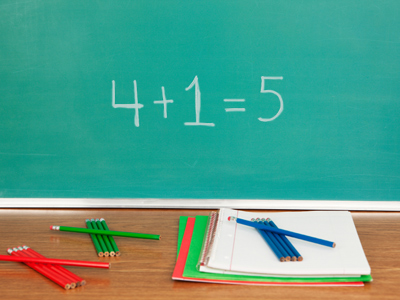To check if 4 + 1 = 5 you could try seeing if 5 - 1 = 4 or 5 - 4 = 1.

# Year 2 Calculation - Inverse Operations

This quiz addresses the requirements of the National Curriculum KS1 Maths and Numeracy for children aged 6 and 7 in year 2. Specifically this quiz is aimed at the section dealing with checking calculations using the inverse operation.

In order to check a calculation, it is useful to know the inverse (or opposite) operation. For example, the inverse operation of addition is subtraction so to check 5 + 6 = 11, you could try 11 - 6 = 5 or 11 - 5 = 6. Another way of checking an addition calculation would be to add the numbers up in a different order - this of course does not work for subtraction!

Question 1
How could you check 62 + 38 = 100?
By taking away 100
By finding the difference between 62 and 38
By subtracting 100 from 62
By subtracting 38 from 100
If 62 + 38 = 100 is correct, then 100 - 38 should give an answer of 62
Question 2
Which calculation could you use to check that 16 - 9 = 7 is correct?
16 + 9 = 7
9 + 7 = 16
7 - 16 = 9
7 - 9 = 16
If 9 subtracted from 16 leaves 7, then by adding the 9 back again, you should be back to where you started
Question 3
How would you check 45 - 23 = 22?
By subtracting 22 from 23
By finding the total of all the numbers
Adding the two smaller numbers should give the larger number
Question 4
If 3 + 4 + 10 + 3 = 20, what could you do to check the answer?
Take out all the larger numbers
Use multiplication
Add some extra numbers in too
Add the numbers up again in a different order:
10 + 3 + 3 + 4 or 10 + 4 + 3 + 3
Addition can be done in any order
Question 5
Use number pairs, or bonds, to 20 to find the incorrect calculation:
20 - 13 = 7
20 - 8 = 12
20 - 16 = 14
20 - 2 = 18
16 + 14 gives a total of 30, not 20
Question 6
Use number pairs, or bonds, to 20 to find the incorrect calculation:
20 - 10 = 20
20 - 0 = 20
20 - 15 = 5
20 - 19 = 1
20 + 10 would not give a total of 20
Question 7
What does 'inverse operation' mean?
Doing the calculation upside down
Making a mistake on purpose
The opposite operation
A medical procedure
The inverse of subtraction is addition, the inverse of addition is subtraction
Question 8
Which calculation could you use to check that 15 + 8 = 23 is correct?
23 + 8 = 15
23 + 15 = 8
15 - 8 = 23
23 - 8 = 15
If a group of 15 and a group of 8 are combined, taking one group away again should leave you with the other
Question 9
How do you know that 20 - 10 = 10?
Because I know 10 + 10 = 20
Because it's a subtraction
Using an addition fact can help to solve a subtraction
Question 10
If 29 - 15 = 14, what could you do to check the answer?
Subtract the numbers in a different order